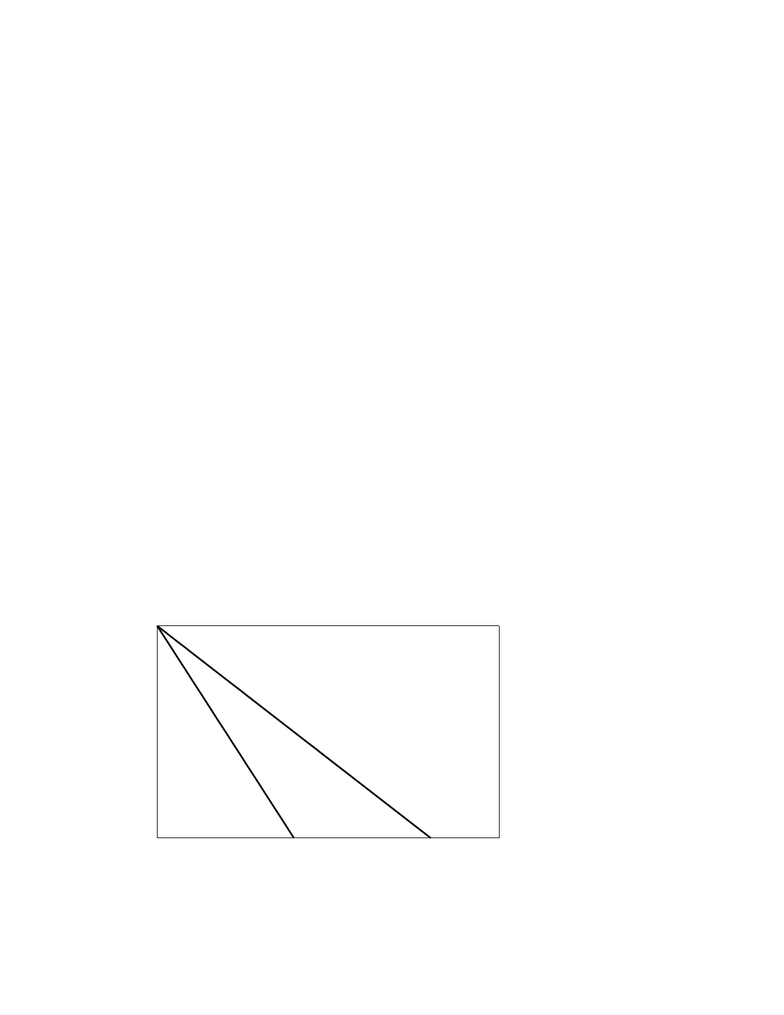Class Notes (1,100,000)
CA (620,000)
York (40,000)
ACTG (300)
Lecture

# monopoly eco

Department
Accounting
Course Code
ACTG 2011
Professor
Eytan Lasry

This preview shows pages 1-3. to view the full 10 pages of the document.Principles of Economics: Monopoly
MONOPOLY
A monopoly (‘single sellers’) is the sole producer in an industry. This means that Demand
for the industry is Demand for the Monopoly and that the marginal cost and average cost functions
of the monopoly are the marginal cost and average cost functions for the industry. A monopoly is
not a price taker since a change in industry output changes the price of the commodity for the
monopoly. . Since price is not constant, marginal revenue is not equal to price for a monopolist.
The Marginal Revenue at a given output is always less than price at that quantity since Marginal
revenue (MR) = P + QP/Q (I derived this formula for marginal revenue in an earlier lecture.)
and P/Q < 0. (Marginal Revenue only equals Price for a monopolist if market demand is
perfectly elastic (horizontal), which is analogous to the perfect elastic demand function facing the
individual competitive firm) Since P is not constant and price is dependent upon the output of the
monopoly, a monopoly can determine commodity price by restricting output. The marginal cost
function of the monopoly is not the supply function for the industry because quantity supplied is
not simply determined by marginal cost in response to a price but depends upon the the
monopolist’s determination of price to maximize profit.
Profit Maximization for a Monopolist: MR = MC
Profit maximization for the monopolist occurs at the output where Marginal Cost = Marginal
Revenue (MC = MR) as we derived earlier for all firms. We cannot simplify this to P = MC
because MR ≠ P.
Deriving Marginal Revenue from Demand: SlopeMR = 2*SlopeD for Linear Demand
We know that Marginal Revenue = P + QP/Q but we can also express Marginal Revenue
in relation to the Demand function. In particular, Linear Demand functions generate linear
Marginal Revenues functions that have twice the slope of the Demand function.
- 1 -

Only pages 1-3 are available for preview. Some parts have been intentionally blurred.Principles of Economics: Monopoly
Proof: A Linear Demand function is of the form P = a – bQ.
=> MR = TR/Q = (P*Q)/Q = (a – bQ)Q/Q = (a – bQ2)/Q = a – 2bQ
(MR = dTR/dQ = d(PQ)/dQ = d(a – bQ)Q/dQ = d(a – bQ2)/dQ = a – 2bQ)
i.e. Marginal Revenue for Linear Demand has the same intercept term as the Demand function
and twice the slope.
We can also say that quantity that gives a specific Marginal Revenue is half the quantity from
the Demand function that gives a Price equal to that Marginal Revenue.
Proof: If Demand is P = a – bQD so that Marginal Revenue is MR = a – 2bQMR, then
P = MR => a – bQD = a – 2bQMR, => bQD = 2bQMR
We already know this by the way from our understanding of the elasticity of a linear Demand
function. Elasticity is unit elastic at the midpoint of a Demand function, which means that
Marginal Revenue is 0 at the midpoint (bQD = 2QMR) because Total Revenue doesn’t change.
E.g. Suppose that Demand is P = 80 – 2Q. What is Marginal Revenue?
Marginal Revenue: MR = 80 – 4Q because slope of Marginal Revenue = 2(-2)
Demand: P = 80 - 2Q = > MR = 80 - 4Q
0 10 20 30 40 50
0
20
40
60
80
P, MR
Q
Demand
MR
MR=0 => unit elasticity
- 2 -

Only pages 1-3 are available for preview. Some parts have been intentionally blurred.Principles of Economics: Monopoly
A monopolist will not produce in the inelastic portion of a Demand function since a decrease
in quantity will a) increase Total Revenue and b) reduce Variable Costs. The monopolist reduces
quantity in the elastic portion of the Demand function so long as the decreased Variable Cost of
one less unit is greater than the decreased revenue from one less unit, i.e., until MR = MC.
Monopoly Equilibrium and Economic Profit
Monopoly Equilibrium => monopoly output (QM) where MR = MC
=> Monopoly Price (PM) from Demand at QM
=> Average Cost (ACM)from Average Cost at QM
=> Economic Profit = (PM – ACM)*QM or PM*QM – Total CostM
E.g. Suppose that P = 80 – 2Q and Total Cost = Q2/2 + 5Q + 200 are the Demand and Total Cost
functions of an Industry.
a) What is the competitive equilibrium price and quantity?
Competitive equilibrium => P = MC
=> 80 – 2Q = Q + 5
Q = 25 and P = \$30 from either 80 – 2*30 or 25 + 5
b) What is the competitive equilibrium economic profit?
Profit = PQ – TC = 30*25 – (252/2 + 5*25 + 200) = \$112.50
c) What is monopoly price and quantity
MR = MC => 80 – 4Q = Q + 5 (Note the slope of MR in relation to Demand)
Q = 15 and P = \$50 from 80 – 2*15
d) What is monopoly profit?
Profit = 50*15 – (152/2 + 5*15 + 200) = \$362.50
This following graph depicts monopoly equilibrium.
- 3 -
###### You're Reading a Preview

Unlock to view full version